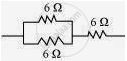# Show how you would connect three resistors, each of resistance 6 Ω, so that the combination has a resistance of 9 Ω. - Science

Numerical

Show how you would connect three resistors, each of resistance 6 Ω, so that the combination has a resistance of 9 Ω.

#### Solution

If we connect the resistors in series, then the equivalent resistance will be the sum of the resistors, i.e., 6 Ω + 6 Ω + 6 Ω = 18 Ω, which is not desired. If we connect the resistors in parallel, then the equivalent resistance will be 6/2 = 3 Ω is also not desired. Hence, we should either connect the two resistors in series or parallel.
Two resistors in parallel:Two 6 Ω resistors are connected in parallel. Their equivalent resistance will be

1/(1/6+1/6)=(6xx6)/(6+6)=3Omega

The third 6 Ω resistor is in series with 3 Ω. Hence, the equivalent resistance of the circuit is 6 Ω+ 3 Ω = 9 Ω.

Concept: System of Resistors - Resistances in Parallel
Is there an error in this question or solution?
Chapter 12: Electricity - Exercises [Page 221]

#### APPEARS IN

NCERT Class 10 Science
Chapter 12 Electricity
Exercises | Q 11.1 | Page 221

Share